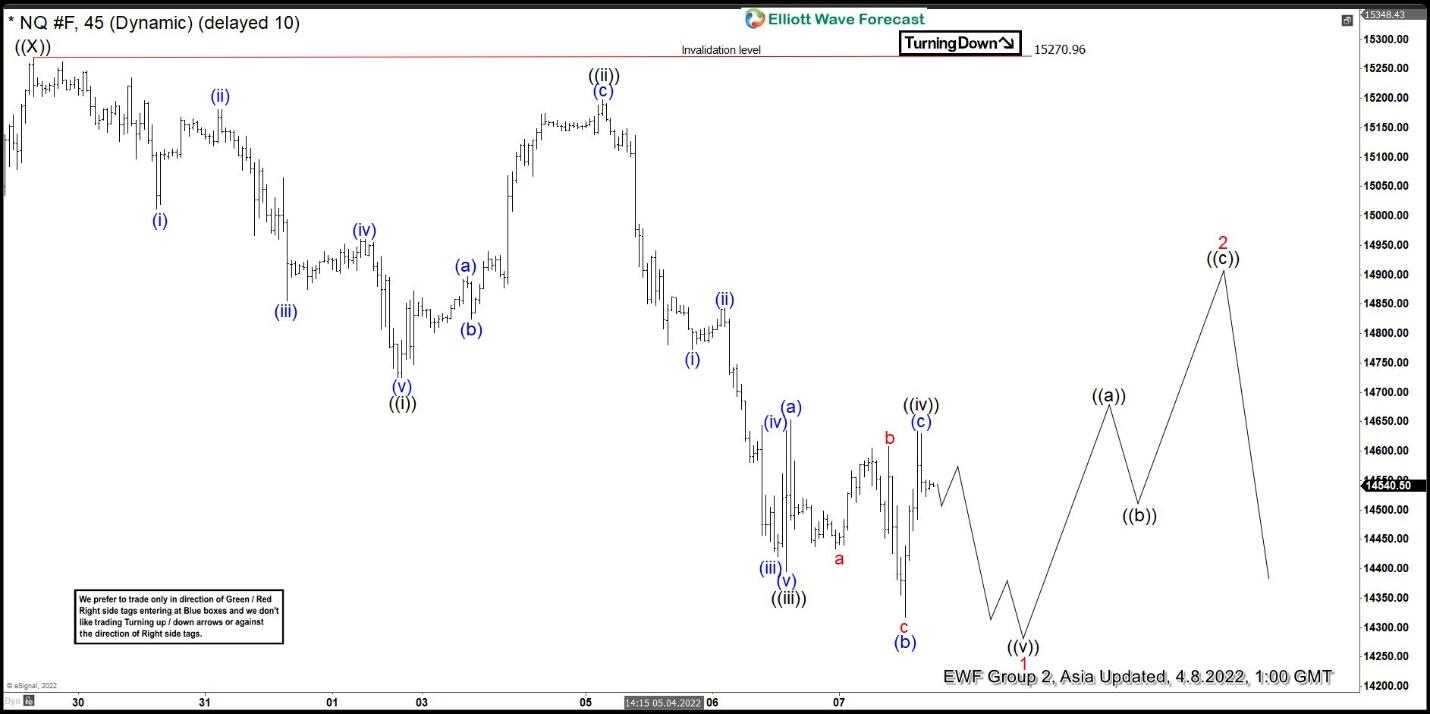Short term outlook in Nasdaq (NQ) suggests the rally to 15270.96 ended wave ((X)). The Index has resumed lower in wave ((Y)), although it still needs to break below the last low on March 15 at 12942.50 to validate the view. The decline from wave ((X)) however looks impulsive suggesting further downside is likely, at least in the near term. Down from wave ((X)), wave (i) ended at 15012.55 and rally in wave (ii) ended at 15180.75. Index then resumes lower in wave (iii) to 14855.50, wave (iv) ended at 14960, and final wave (v) lower ended at 14725 which completed wave ((i)).

Index then corrected in wave ((ii)) towards 15198 before turning lower again. Down from wave ((ii)), wave (i) ended at 14773.50 and wave (ii) ended at 14843. Index then continues lower in wave (iii) to 14420.5, wave (iv) ended at 14634.25, and wave (v) ended at 14394.75 which completed wave ((iii)). Wave ((iv)) correction took the form of an expanded flat where wave (a) ended at 14652.75, wave (b) ended at 14317 and wave (c) ended at 14633.75. Near term, expect the Index to extend lower in wave ((v)) which should complete wave 1 in higher degree. Index should then rally in wave 2 to correct the decline from wave ((X)) before it resumes lower. Near term, as far as pivot at 15270.96 high stays intact, expect rally to fail in the sequence of 3, 7, or 11 swing for more downside.

#### NQ 45 Minutes Elliott Wave Chart#### Nasdaq Elliott Wave Video

[embedded content]